Project

# Build Your Own Time-Domain Reflectometer

October 08, 2015 by Benjamin Crabtree

## Build your own TDR out of a scope and a signal generator to troubleshoot long cable runs and impedance mismatches.

TDRs are expensive, but here's how to make your own for a fraction of the cost!

### What's a TDR?

Time Domain Reflectometry is a powerful technique in which a pulse is generated to propagate down a cable, after which the reflected signal returns to the generator and is then interpreted based on its shape, phase, and delay. The results can be used to determine the length of a cable, if and where there is an open circuit, what kind of load a cable is terminated with, and even the relative permittivity and permeability of a dielectric. TDRs are used in a wide range of applications including aviation and naval craft troubleshooting where there are often miles of cable and a technician can accurately pinpoint a malfunction. They are also used in devices measuring soil moisture content in which a cable is placed in the soil and the relative permittivity (dielectric constant) of the soil can be calculated. Because water has a very high relative permittivity and dry soil does not, the permittivity that is detected will correlate to the amount of moisture present. TDRs are used in this same way as a level indicator for liquids, where a thin probe is submerged and detects lower permittivity as the level drops. Most circuit board verification jigs will implement this technique as a method of determining if a chip on a ball-grid-array solder pad has any open pins.

### How It Works

The physics behind this method can be pretty complex (single equations that take up half a page), but we will work with a few assumptions that will make life easier and still get us a very close answer; in engineering, it is important to not let perfection be the enemy of good enough. Firstly, we will assume we are working with a lossless dielectric, meaning the vector describing this dielectric contains no real component ‘R’ and the loss tangent is 0°. Secondly, we will assume that our cable material (copper) is a perfect conductor and the vector describing it contains no imaginary component “ωj”. The basic principle is that we want to send a short pulse into a cable and use a visualization tool to examine the reflection. When a signal is incident to a cable, the signal does not travel to the end of the cable instantaneously. For starters, the finite speed of light (299,792,458 m/s) is the upper boundary, but as we will soon see, we will not even achieve these speeds; the reason is that the constants of relative permittivity and permeability for our cable's dielectric will slow down transmission speed or phase velocity, Vp. The signal will still be traveling at a significant fraction of the speed of light but keeping this value precise is key to the math coming out right. Phase velocity with our assumptions is defined as

Thus the time it will take for our incident signal to travel to the load can be calculated as

We will then expect to see a delay in our reflected signal corresponding to the results of these equations. We will get into more detailed calculations in a moment, for now, let’s get building

### Implementation

TDRs are expensive, but fortunately, a rudimentary version can be easily assembled using a signal generator and an oscilloscope. In addition to these items you will need a set of BNC connectors and of course, a cable (can be to test it; you may also use a set of precision matched loads as seen in the image below, or you can use a potentiometer connected to the end of the cable, though this method introduces a bit of noise. The matched set I will be using consists of a 50, 75, and 93 Ohm loads.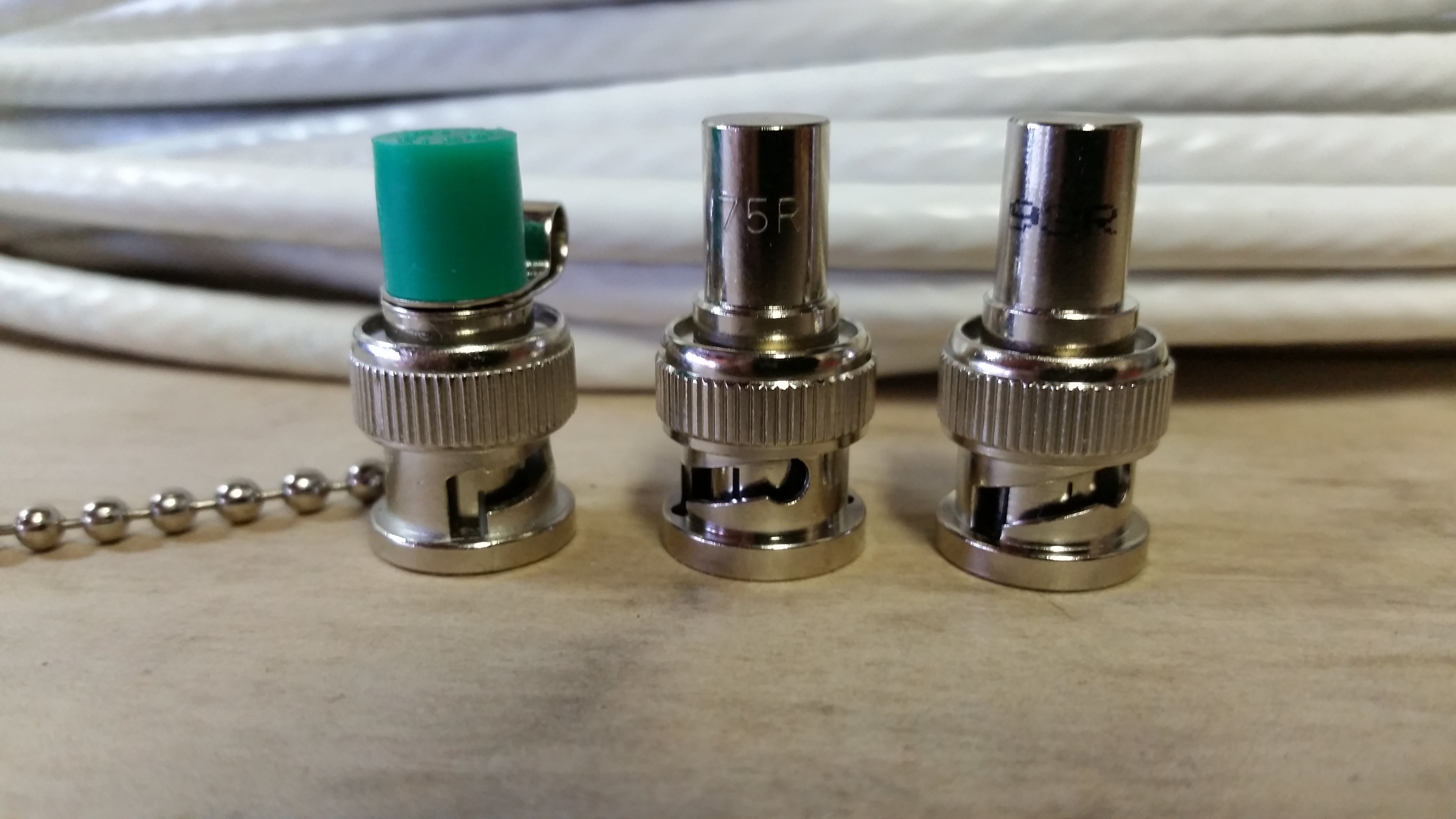One of the most readily available cable available is a piece of coaxial cable. Coaxial cable has been around for a very long time, but it has improved in construction significant over the years. Any coaxial cable will have a characteristic impedance partly determined by the diameter of the core cable that corresponds to its intended usage; they will range between 30 Ohms to 93 Ohms, with 30 ohms having the highest power handling capacity, and 77 ohms having the least signal attenuation. 50 Ohms is a common coaxial cable impedance as it is a compromise between power handling and signal loss. For this test I will use a long piece of coax cable, but I won’t say just yet how long it is or what its characteristic impedance is yet- that’s what we are here to find out! I will tell you that the manufacturer lists its relative permittivity as 1.2. For all coaxial dielectrics, the relative permeability is so close to 1 that we can just assume it is for our purposes ( accurate to within 0.00000001%). Substituting these values into our first equation we get

$$V_{P} = \text{273, 671, 819.7 m/s or 10.7745 in/nS}$$

which means our signal will propagate at about 91.29% of the speed of light, this percentage is known as the velocity factor or VF. Now we will hook up our gear and see what we get. Connect the signal generator to your scope with a T connector and the shortest cable you can find, this will help minimize error and the T will give you a place to connect the coaxial mystery cable- seen in the image as a white cable.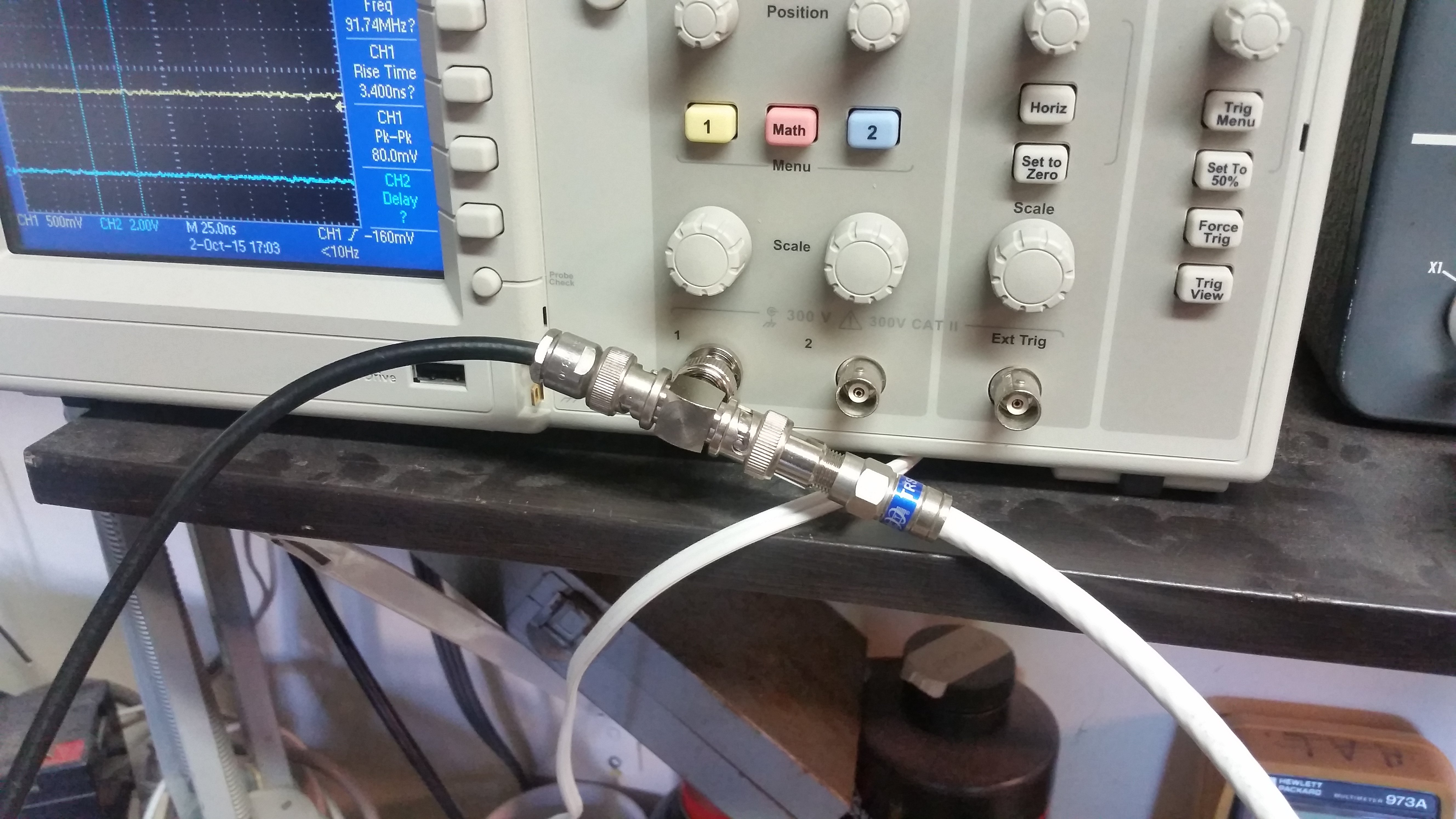### Open Circuit Configuration

After connecting one end of the coaxial cable to the T connector, leave the other end open; this first test will be an open circuit test. The open circuit test will give a very good representation of delay time and reflection coefficient. The frequency that you the signal generator to is arbitrary, but it works best if you have a really fast rise time. In my case, I am connecting to the 'Pulse Out' terminal that can be seen in the image below.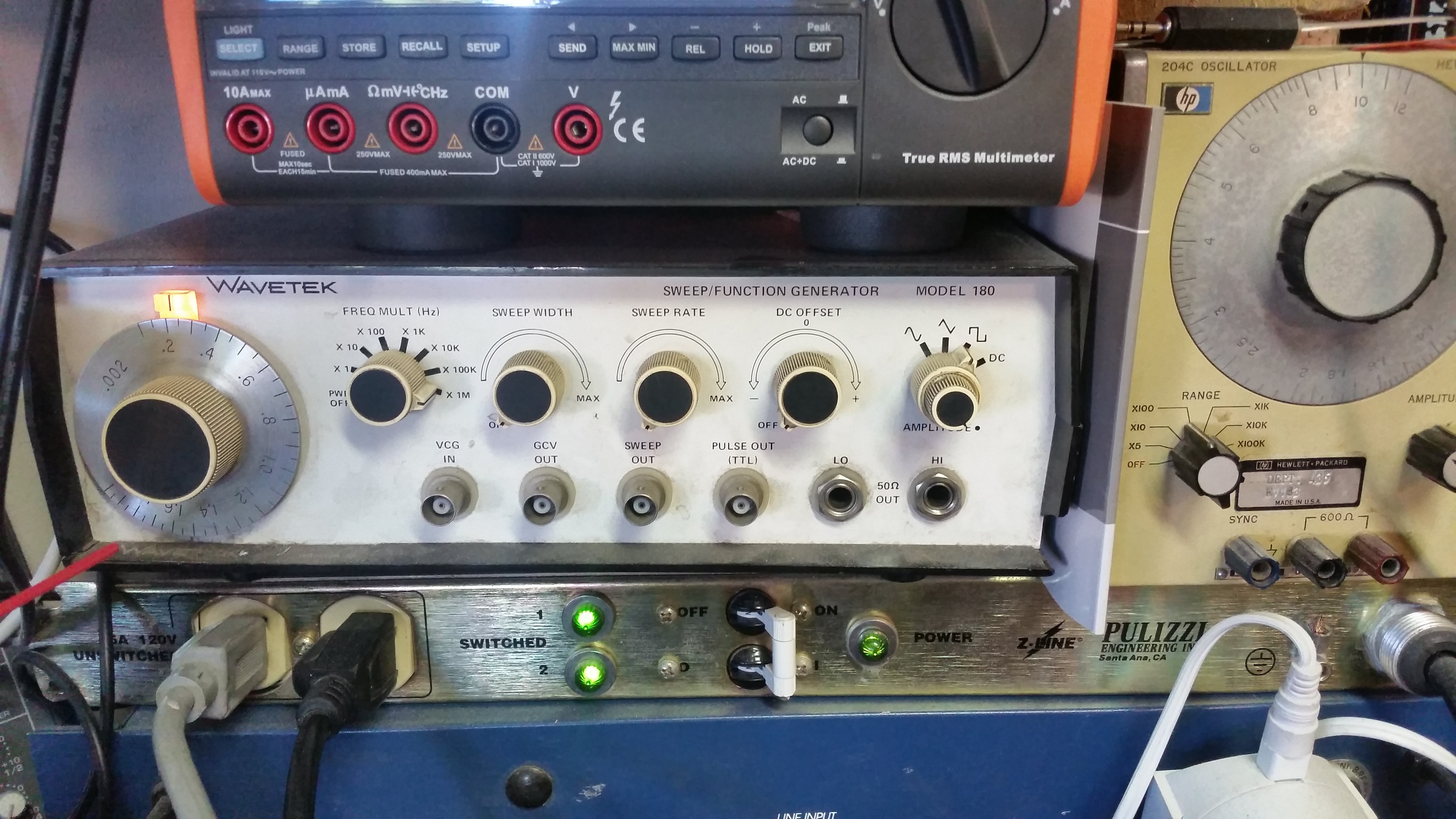With the signal generator hooked up, trigger the scope and you should see an image like the one below: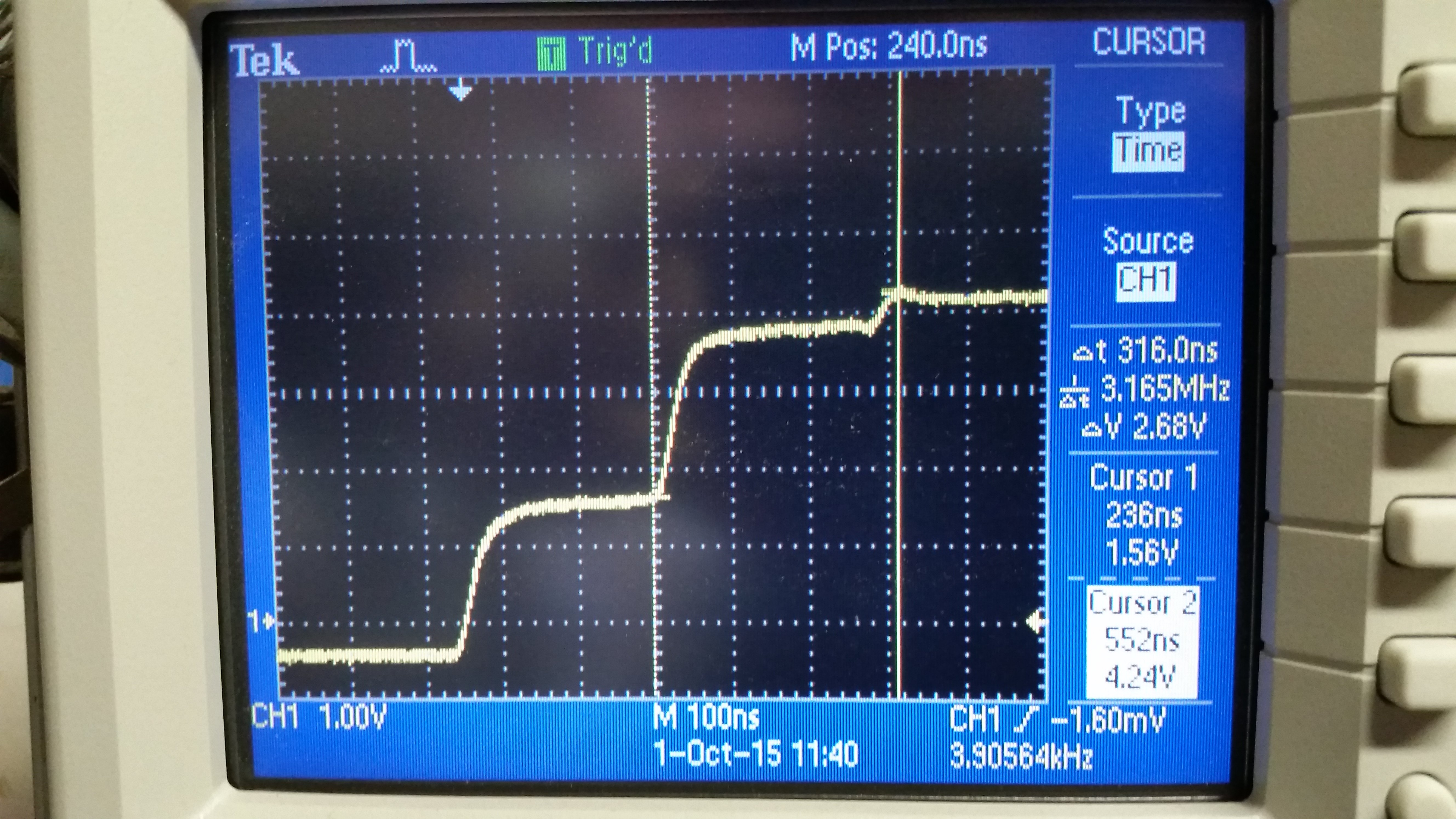Here we see our incident wave as the bottom step with the reflected wave as the top step. Because there is a finite transit time (calculated earlier) in the line, its input impedance will look like the characteristic impedance of the line for   so the line appears to be infinitely long until the pulse has had a chance to traverse the cable and reflect back to the input. the pulse begins as V0/2 because it is expecting the input impedance and load to be matched, creating a voltage divider. In the open circuit case, the entire signal is reflected back towards the generator as can be seen using the equation for the reflection coefficient

giving us a coefficient of 1 for the load being infinite. After reflecting back, the signal jumps back up to V0, in this case about 4.5-5 volts. Let's take a closer look at what is going on: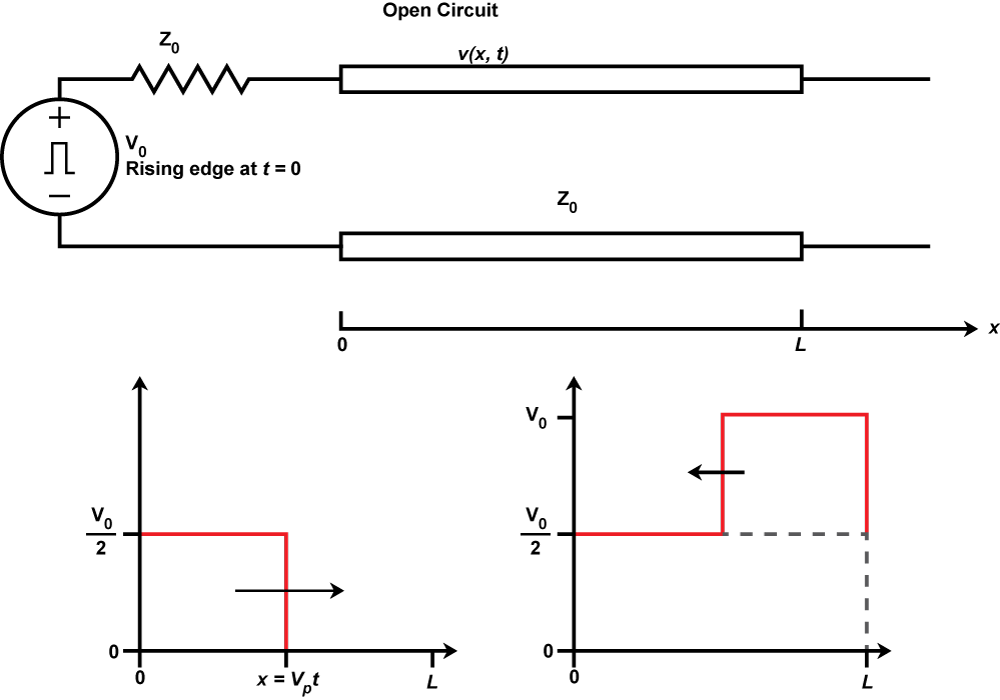The diagram shows that the leading edge of the pulse will be at position x at  and the reflected signal is added to the incident signal since the reflection coefficient is positive. The signal does not become reflected a second time because it is terminated by the output impedance of the generator. Using the cursors of the scope, we can measure the delay; alternatively, if we hook up the open circuit end of the cable up to channel 2 of the scope (still essentially an open circuit) we can have the scope measure the delay for us, giving a more accurate result. The image below shows a delay of 111-112 nS. For our calculations, we will use 111.5 to average it out.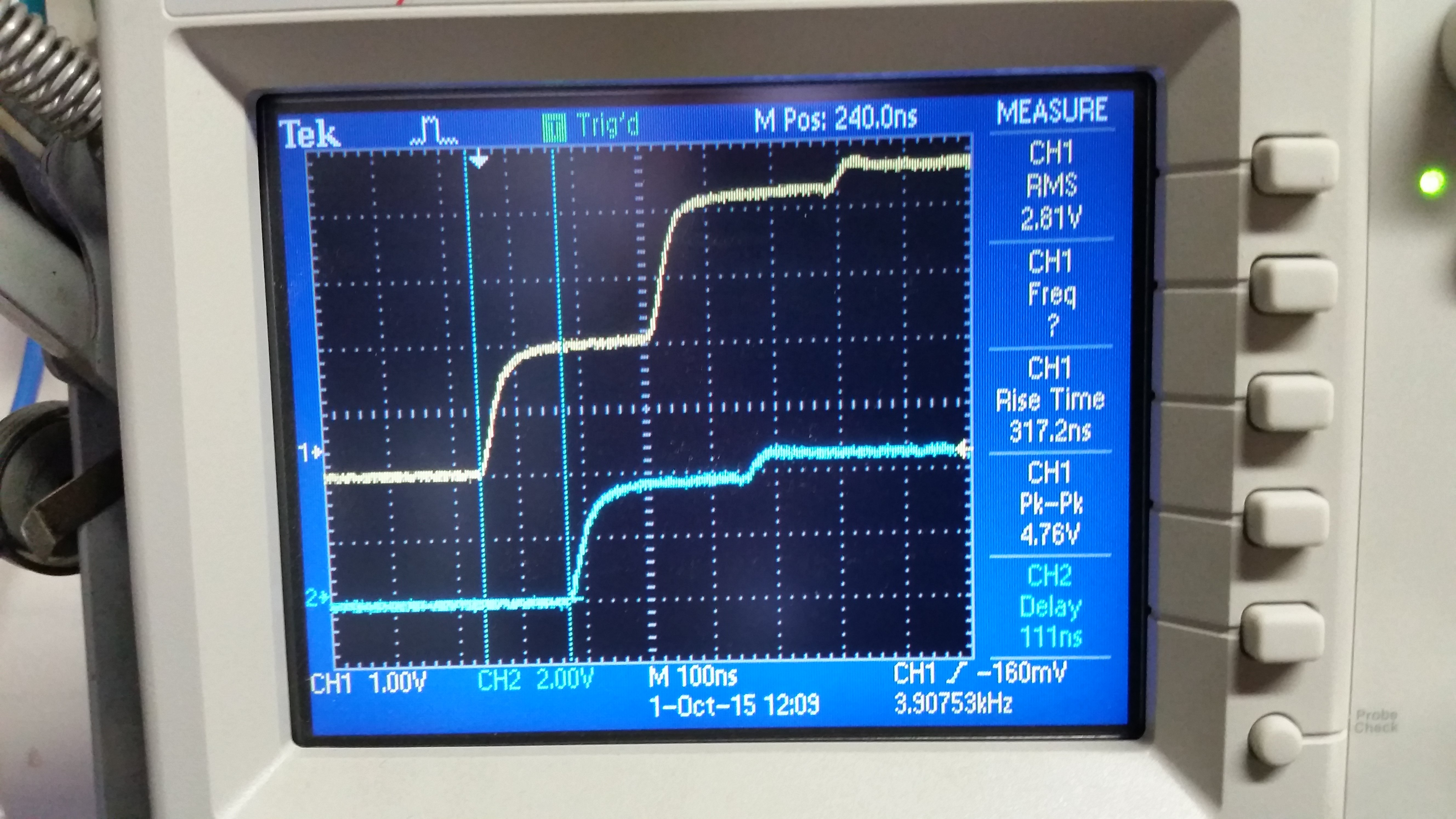Using our previous value for Vp, we can now calculate the length of our cable:

Indeed, this is a 100-foot long coaxial cable (though twisted-pair cables will also work), and our result is very close, discrepancies may have come from the addition of the connectors or the cable length being slightly out of spec; either way, it demonstrates that this technique can be very accurate. This method is how a military technician could locate an open circuit on an aircraft carrier will hundreds of miles of cable to sort through, it is easy to see how this could really save time. Earlier I mentioned that the reflected wave will not reflect a second time due to the output impedance of the signal generator, which is true in the theoretical case where the signal generator has the same impedance as the characteristic impedance; in our measured case, we see something different- see the small third step on the yellow trace? That tells us that the generator impedance is not matched to the characteristic impedance. In this case, my generator has a 50 Ohm output impedance, the results tell us that this coaxial cable must not be a 50 Ohm line.

### Short Circuit Configuration

Now disconnect the channel 2 end of the cable and short it to the shield with a small piece of wire, the results should look similar to the below scope trace: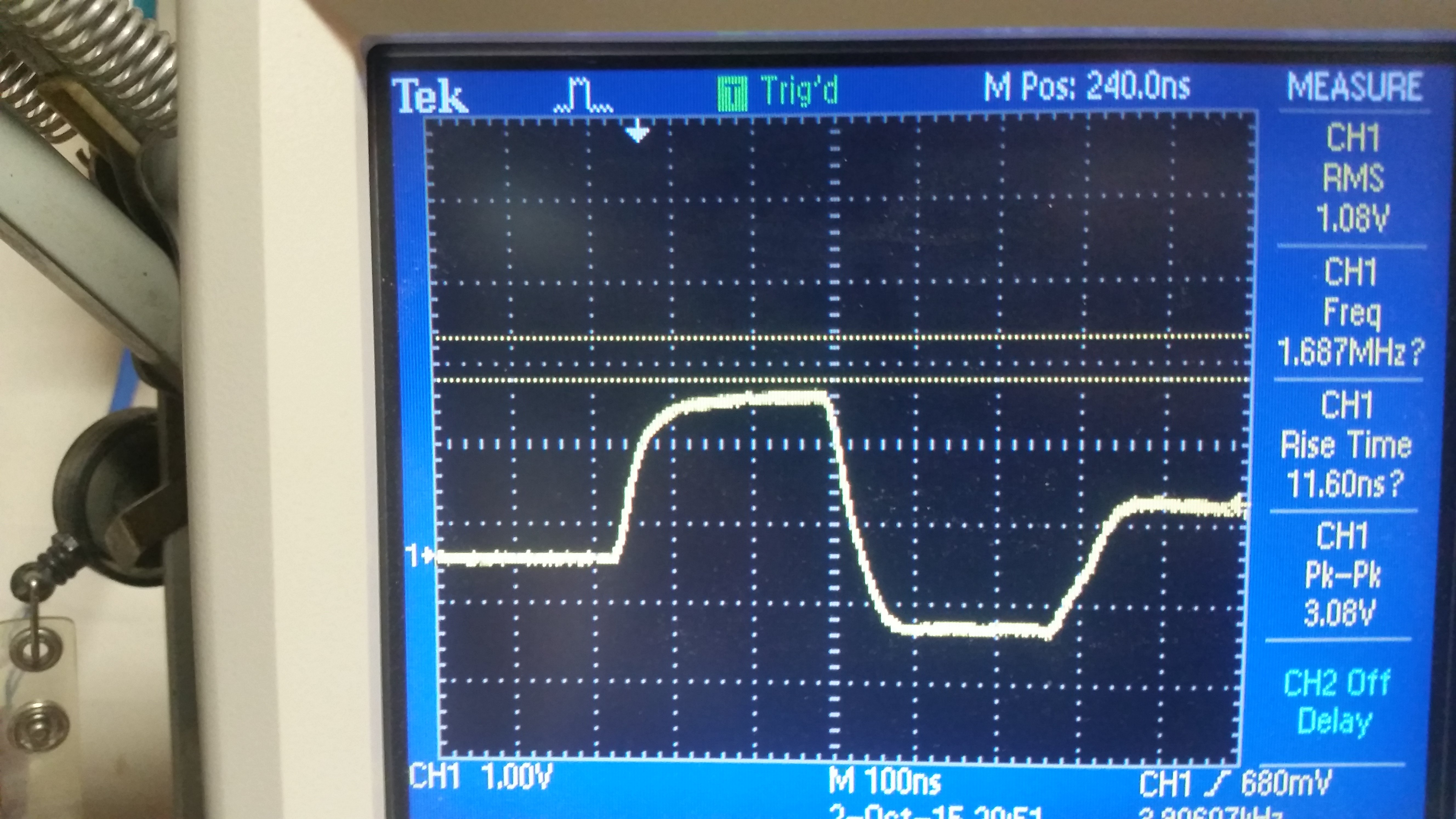So we have our pulse propagate through the cable and then get canceled out. This can be hard to demonstrate in real life because electronic devices (signal generators included) do not like to see short circuits. To see what is really taking place, we will turn to our model.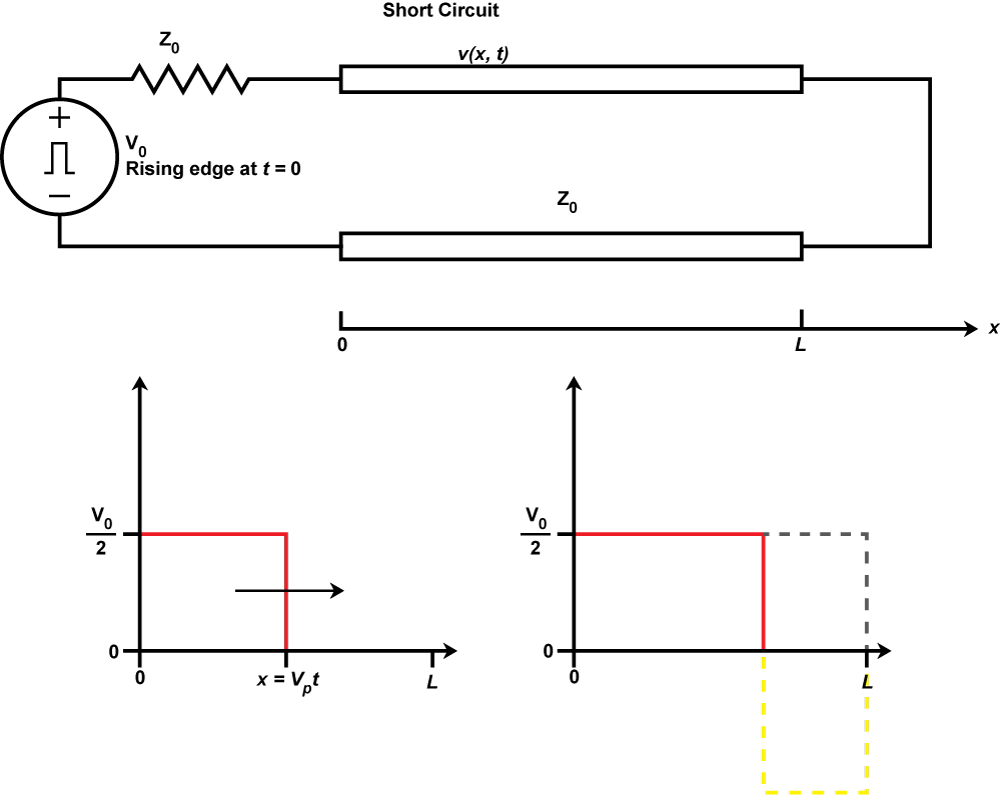When the signal begins to propagate, the input impedance appears to be Z0 and the pulse has an amplitude of V0/2 but the short circuit load has a reflection coefficient of -1 from our equation

which has the effect of inverting the reflected signal and phase canceling the rest of the incident wave for all time where  after which the circuit is in a steady state where all voltages are zero. In practice, this technique can be used to generate extremely short pulses, shorter than the hardware would normally allow.

Next, we will hook up the 75 Ohm load, we know that the characteristic impedance is not 50, and 75 is the next most likely candidate. Upon hooking up the load the scope displays the following: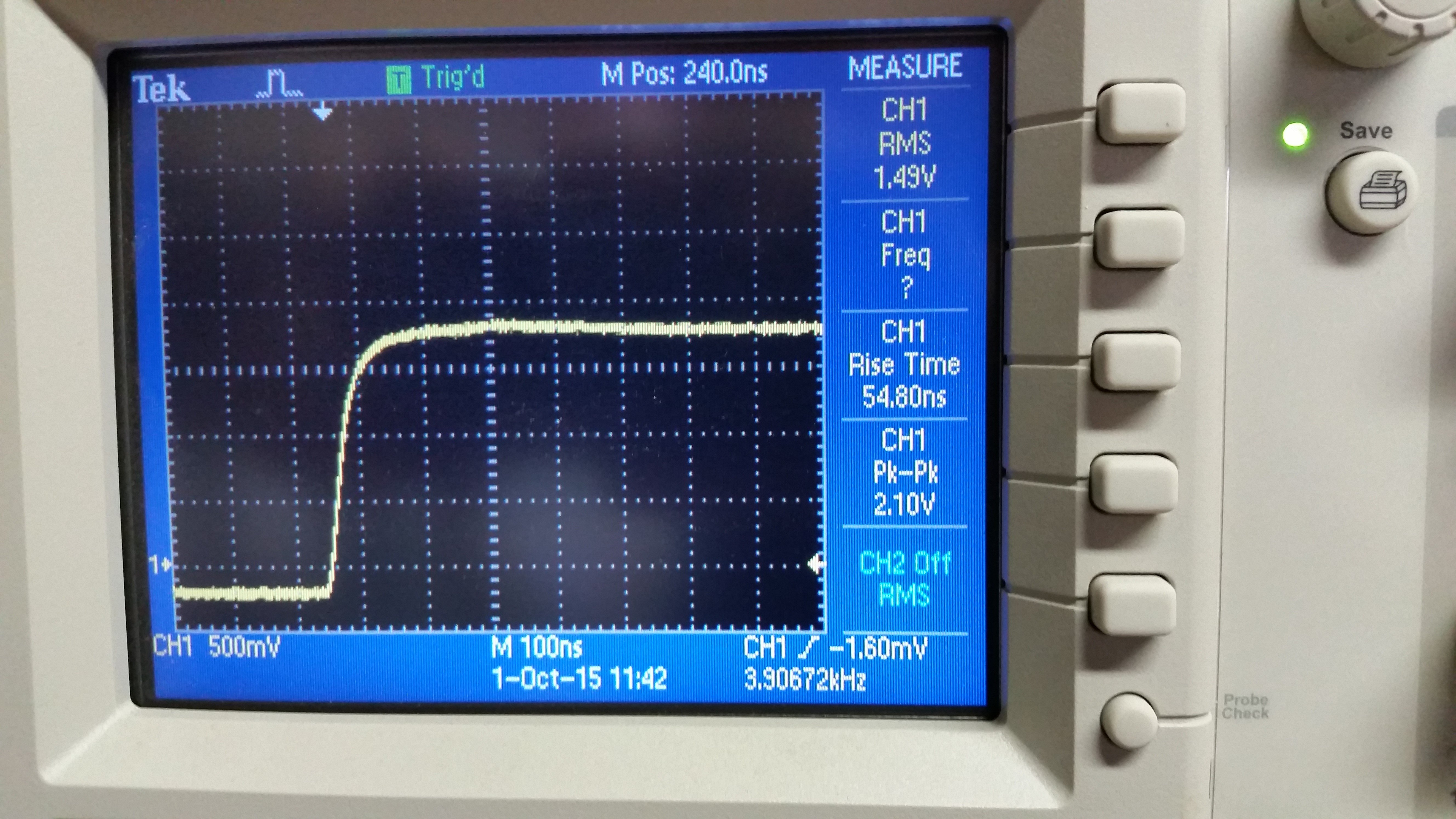The trace is a clean pulse with a magnitude of  about V0/2 which tells us that our line is matched, thus we now know that this cable is a 100 foot long 75 Ohm coaxial cable, which means it is most likely designed for cable TV installations as very little power handling is required and signal loss must be minimized. The model of what is going on is below: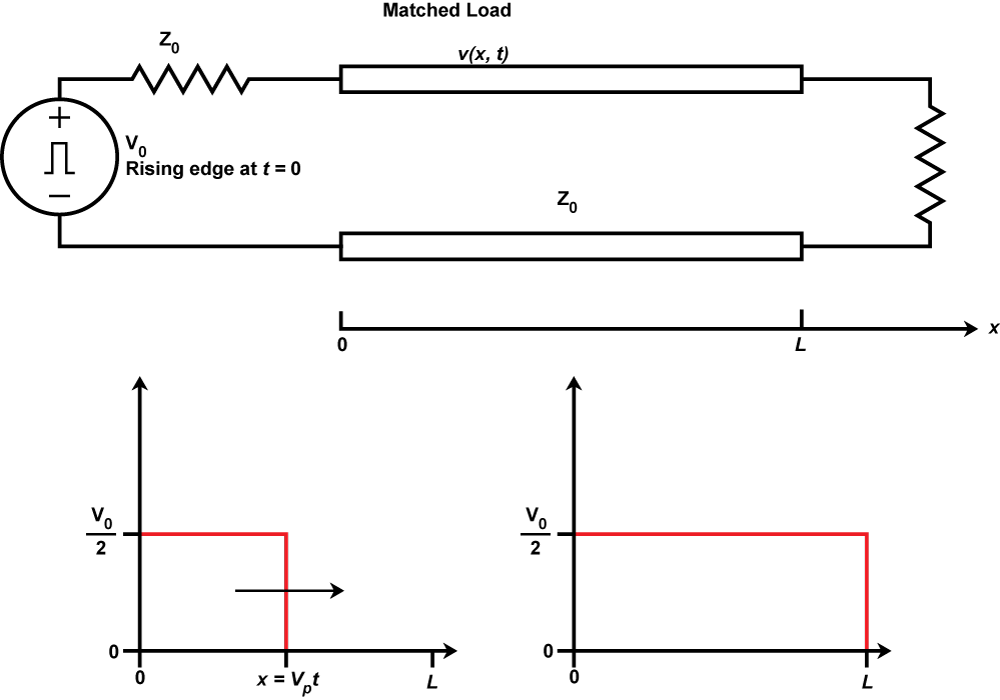The incident wave reaches the load at  but since the load is matched to the line there is no reflection (reflection coefficient = 0) and the circuit acts as a voltage divider giving us half the magnitude of our incident wave.

Finally we will use the precision loads to simulate a mismatched load condition. First we will hook up the 93 Ohm load to the end of our cable and the scope displays the following: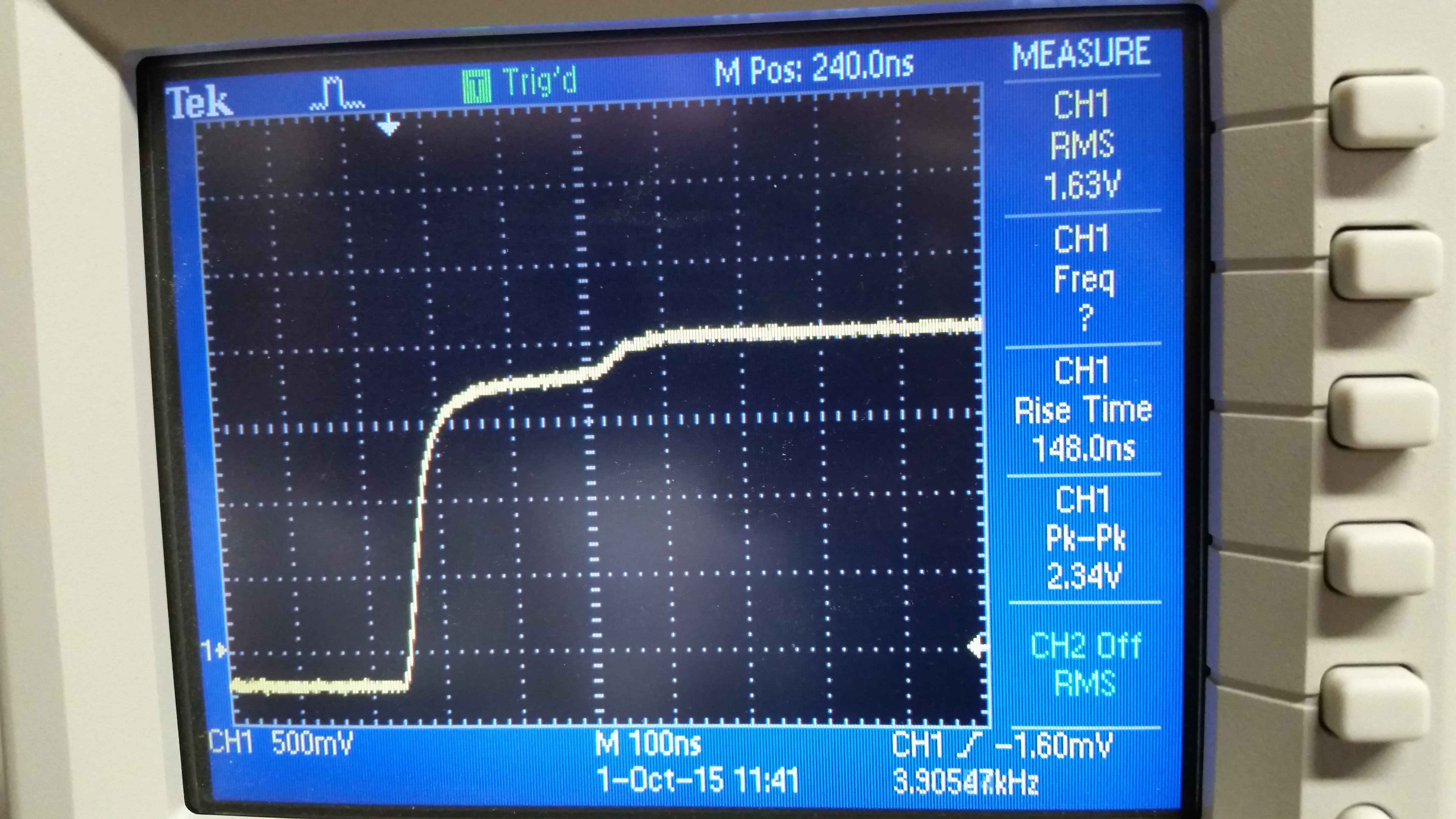The scope shows us that we have a slight mismatch, but how much? Now that we know our Characteristic impedance, we can calculate the reflection coefficient for an arbitrary load.

Thus about 10.7% of the incident wave is not being absorbed by the load due to mismatch, appearing on the scope as a reflection informing us that the load is too large, as indicated by the positive polarity of our reflection coefficient. If we then hook up the 50 Ohm load, we can see an example of a load that is to small: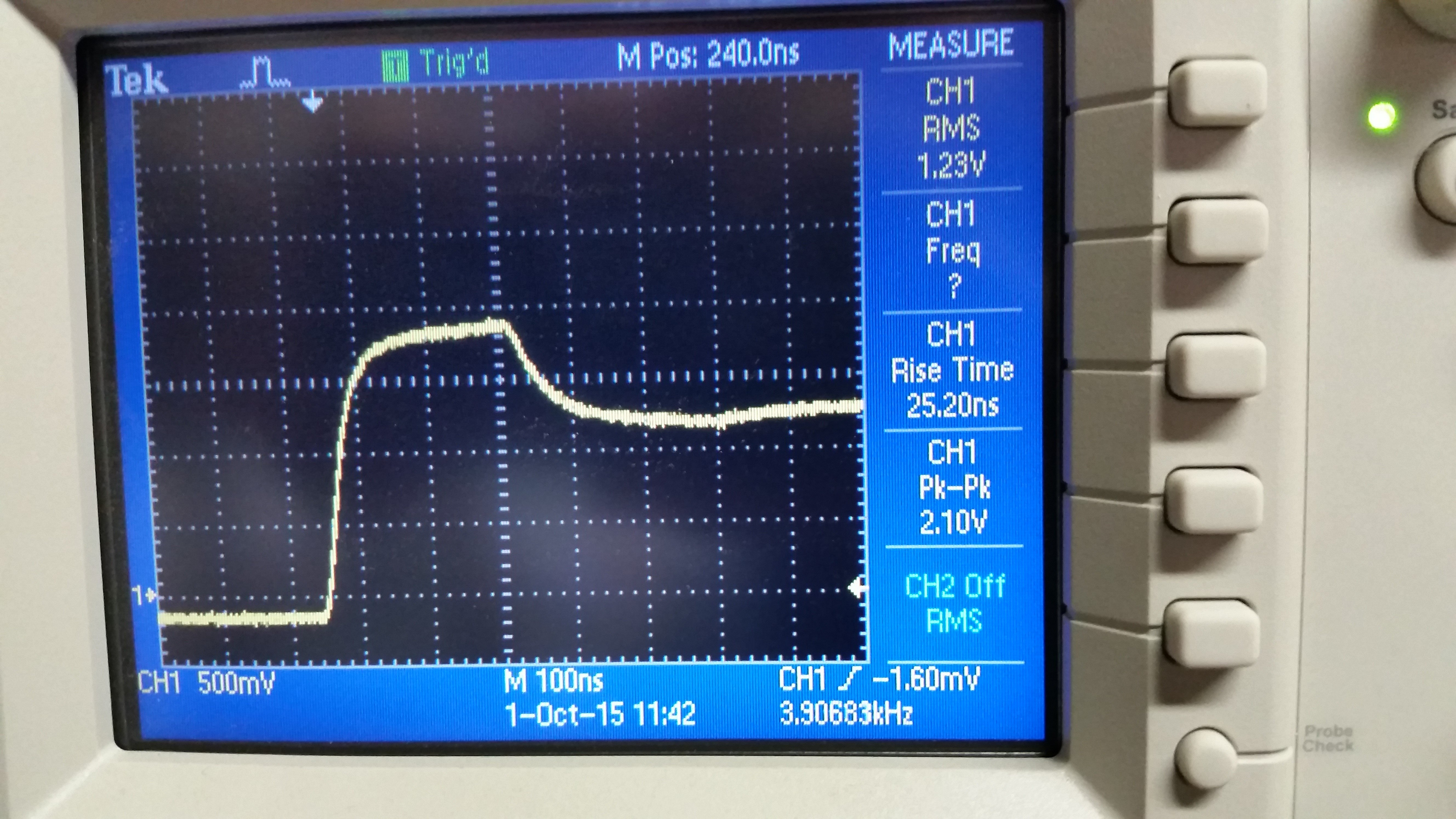Now we have part of our wave being canceled by the small load, this is a hint that our reflection coefficient will be negative.

Sure enough, the math tells us that 20% of our wave is going to be phase canceled as indicated by the negative sign. Both of these mismatched conditions can cause severe signal degradation, and it is important to ensure proper matching when working with cables. TDRs are powerful troubleshooting tools and being able to make one out of standard bench equipment and interpret the results can help the emerging engineer gain mastery over transmission line theory. With as prevalent as these devices are, it is likely that every engineer will end up using one at some point for any number of applications. On the other hand, you can always just use it to detect if your landline has been wire-tapped, but we will leave that up to the discretion of the reader.• M
MattWCarp October 10, 2015

The equation for the reflection coefficient in the case of the short circuit is actually the case of the open circuit.

Can someone explain this comment and formula:

“Because there is a finite transit time (calculated earlier) in the line, its input impedance will look like the characteristic impedance of the line for t > 2l / Vp ”      I don’t see where 2l comes in.  Of course, I totally understand that t * Vp is x

Otherwise, great article!

Like.
•Umberto Ravaioli October 16, 2015

This is a transient case, so the standard transmission line steady-state analysis (complex functions for frequency domain) does not apply.  The input will not know that the line is not matched properly (even with a proper termination, the issue generating the reflection cause a mismatch)  until the signal is received back at the generator.  2L is the distance for the signal to go back and forth to the input again.  If one works with resistive impedances, then the algebra as given is valid, even if it is expressed in terms of impedances which can be complex function and therefore only defined for a steady state (frequency domain) situation, not for a transient which works in the time-domain and is analyzed with real function.  If the line had high losses and a complex characteristic impedance things would not work out as well.

You may get some more details at

Also, you are correct about the formula for the reflection coefficient being written for the open case, although the text indicates correctly that the reflection coefficient for a short is -1.  It must be a cut and paste mistake (although, the math in that equation is naive because you do not take limits to infinity that way.  Fortunately it is a simple fraction, not so lucky with more complicated functions).

Like.
• R
refelectronics October 16, 2015

@MattW

Right the equation for short ckt s/b (0-Z0)/(0+Z0) = -1, the wave is 100% reflected and inverted.  So I think the quote:

“Because there is a finite transit time (calculated earlier) in the line, its input impedance will look like the characteristic impedance of the line for t > 2l / Vp ”

is just intended to say that the cable starts to look like a simple (ideal) wire after 2 times the wave transit time, where the 2 comes from the fact that it only travels down to the load and back to the source where it is absorbed (so 2x.)  If the cable is not terminated in its characteristic impedance at the source, then the wave will also reflect there and so the time to settle will get longer (as it loses a little of its energy at each reflection) and that 2 could get a lot bigger.

Like.